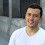Banana Cherry Green Apple | Mathematics Puzzle

Can you solve the above math puzzle? Share your answer in the comment box.

Equation 1: 15 + 15 = 30  (So, 1 Banana = 15)
Equation 2: 10 + 10 = 20  (So, 2 Cherries = 10
Equation 3: 4 + 4 = 8  (So, 1 Green Apple = 4)
Equation 4: 15 + 5 x 4  (Here 1 Banana = 15 ; There is only 1 cherry = 5 ; 1 Green Apple = 4)
Using BODMAS rule, calculate multiplication before addition, So the equation becoms:

=> 15 + (5 x 4)
=> 15 + 20
=> 35

1.15+(5*4)=
15+20=35

2.If you have noticed, this is just all about doing the reverse of what we did a while ago to convert ounces to kilograms. Fortunately, this kg to oz things as well can be reversed to the oz to kg conversion. The whole thing is fun and much simpler than you think. mm to inches

3.This comment has been removed by the author.